• 线性回归模型
例子：基于广告数据理解线性回归模型

销售额与广告预算之间是否有关系
相关性的强度
哪一种媒介对销售额的贡献最大
媒介对销售额影响估计的精确度
未来销售额预测的精确度
该关系是否是线性相关的
广告媒介是否有协同作用

简单的线性回归模型

定义线性回归模型：

Y≈β0+β1X Y \approx \beta_0 +\beta_1 X
β0和β1称为总体参数$\beta_0和\beta_1称为总体参数$
β^0和β^1$\hat\beta_0和\hat\beta_1$为模型参数，通过训练集学习产生
估计得回归方程：Y^=β^0+β^1X \hat Y = \hat\beta_0 +\hat\beta_1 X

参数估计

拟合方法：least squares fit

SSE=e21+e22+...+e2nSSE = e_1^2 + e_2^2 + ... +e_n^2e2i=(yi−y^i)2e_i^2 = (y_i - \hat y_i)^2
目标：SSE（误差平方和）最小化
解：
β^1=∑n1(xi−x¯)(yi−y¯)∑n1(xi−x¯)\hat\beta_1= \frac{\sum_1^n(x_i-\overline{x})(y_i-\overline{y})} {\sum_1^n(x_i-\overline{x})}β^0=y¯−β^1x¯\hat\beta_0=\overline y - \hat\beta_1 \overline x

参数估计评价-模型的假定

确定假定的模型是否合理：要对变量之间的关系的显著性进行检验。

X和Y真正的关系为：Y=f(X)+ϵ$Y = f(X)+\epsilon$.f$f$是某个未知的函数，ϵ$\epsilon$是个均值为零的随机错误项。回归分析中的显著性检验是以对误差项ϵ$\epsilon$的下列假设为依据和进行的：
1. E(ϵ)=0$E(\epsilon) = 0$,意味着β0;β1$\beta_0;\beta_1$都是常数
2. 对于所有的x值，ϵ$\epsilon$的方差相同，用σ2$\sigma_2$表示
3. ϵ$\epsilon$相对独立
4. 误差项ϵ$\epsilon$是一个正态分布的随机变量

假设ϵ$\epsilon$与X独立.评价总回归线与最小二乘线之间的差别.相当于标准统计学中利用样本预测总体的方法。样本均值与总体均值不同，但通常样本均值对总体均值提供了一个较好的估计。同理，β0和β1$\beta_0和\beta_1$在现实中是未知的，我们试图利用β^0和β^1$\hat\beta_0和\hat\beta_1$来估计。

通过计算β^0和β^1$\hat\beta_0和\hat\beta_1$的标准差来评价与真实参数的距离：

SE(β^0)2=σ2[1n+x¯2∑n1(xi−x¯)2]SE(\hat\beta_0)^2=\sigma^2[\frac {1}{n} + \frac {\overline x^2} {\sum_1^n(x_i-\overline x)^2}]SE(β^1)2=σ2∑n1(xi−x¯)2SE(\hat\beta_1)^2 = \frac {\sigma^2} {\sum_1^n (x_i-\overline x)^2}σ2=Var(ϵ)\sigma^2 = Var(\epsilon)
通常，σ2$\sigma^2$是ϵ$\epsilon$的方差，从回归模型和它的假设中可以得出结论：σ2$\sigma^2$也是因变量y关于回归直线的方差，SSE是实际观测值关于估计得回归直线变异性的度量，用SSE除以它的自由度，得到均方误差。均方误差给出了σ2$\sigma^2$的一个估计量（每个平方和都有一个与之相关联的数，这个数叫做自由度，为了计算SSE，必须估计两个参数β0;β1$\beta_0 ;\beta_1$，所以SSE的自由度是n-2）s=MSE−−−−−√=SSE/(n−2)−−−−−−−−−−−√s=\sqrt {MSE} = \sqrt {SSE/(n-2)}
MSE是均方误差，s为估计得标准误差
95%置信区间：[β^1−2SE(β^1),β^1+SE(β^1)][\hat\beta_1-2SE(\hat\beta_1),\hat\beta_1+SE(\hat\beta_1)]
通过SE(β^1)$SE(\hat\beta_1)$还能够进行假设检验，计算出p值和t值，进一步评估参数估计得准确性。t=β^1−0SE(β^1)t = \frac{\hat\beta_1-0} {SE(\hat\beta_1)}

##评价模型的精确度

MSEMSE
R2$R^2$称为判定系数，理解为总平方和中能被估计得回归方程解释的百分比
SST=∑(yi−y¯)SST = \sum(y_i-\overline y )
TSS表示利用均值来估计所产生的离差平方和，称为总的平方和
SSR=∑(y^i−y¯)2SSR = \sum(\hat y_i - \overline y)^2
SSR 称为回归平方和，用于度量y^和y¯$\hat y 和 \overline y$之间的偏离程度
SST=SSR+SSESST = SSR + SSE
利用三个平方和能够给出回归方程一个拟合优度的度量

###多元线性回归

Y≈β0+β1X1+...βnXn Y \approx \beta_0 +\beta_1 X_1+...\beta_n X_n

展开全文• 一般线性模型和线性回归模型Interpretability is one of the biggest challenges in machine learning. A model has more interpretability than another one if its decisions are easier for a human to ...
一般线性模型和线性回归模型Interpretability is one of the biggest challenges in machine learning. A model has more interpretability than another one if its decisions are easier for a human to comprehend. Some models are so complex and are internally structured in such a way that it’s almost impossible to understand how they reached their final results. These black boxes seem to break the association between raw data and final output, since several processes happen in between. 可解释性是机器学习中最大的挑战之一。 如果一个模型的决策更容易让人理解，那么它的解释性就会比另一个模型高。 有些模型是如此复杂，并且内部结构如此复杂，以至于几乎无法理解它们是如何达到最终结果的。 这些黑匣子似乎打破了原始数据和最终输出之间的关联，因为它们之间发生了多个过程。
But in the universe of machine learning algorithms, some models are more transparent than others. Decision Trees are definitely one of them, and Linear Regression models are another one. Their simplicity and straightforward approach turns them into an ideal tool to approach different problems. Let’s see how. 但是在机器学习算法领域，某些模型比其他模型更透明。 决策树绝对是其中之一，而线性回归模型又是其中之一。 它们的简单和直接的方法使它们成为解决不同问题的理想工具。 让我们看看如何。
You can use Linear Regression models to analyze how salaries in a given place depend on features like experience, level of education, role, city they work in, and so on. Similarly, you can analyze if real estate prices depend on factors such as their areas, numbers of bedrooms, or distances to the city center. 您可以使用线性回归模型来分析给定地点的薪水如何取决于经验，学历，职位，所工作的城市等特征。 同样，您可以分析房地产价格是否取决于面积，卧室数量或距市中心的距离等因素。
In this post, I’ll focus on Linear Regression models that examine the linear relationship between a dependent variable and one (Simple Linear Regression) or more (Multiple Linear Regression) independent variables. 在本文中，我将重点介绍线性回归模型，该模型研究因变量与一个(简单线性回归)或多个(多个线性回归) 自变量之间的线性关系。
简单线性回归(SLR) (Simple Linear Regression (SLR))
Is the simplest form of Linear Regression used when there is a single input variable (predictor) for the output variable (target): 当输出变量(目标)只有一个输入变量(预测变量)时，是使用线性回归的最简单形式：
The input or predictor variable is the variable that helps predict the value of the output variable. It is commonly referred to as X. 输入或预测变量是有助于预测输出变量值的变量。 通常称为X。 The output or target variable is the variable that we want to predict. It is commonly referred to as y. 输出或目标变量是我们要预测的变量。 通常称为y 。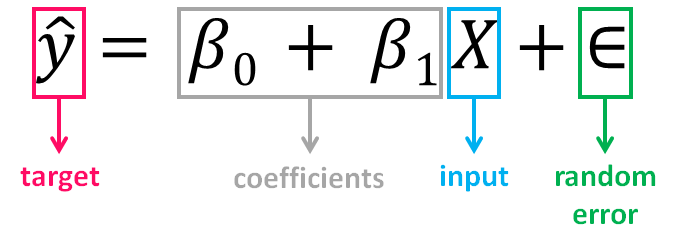The value of β0, also called the intercept, shows the point where the estimated regression line crosses the y axis, while the value of β1 determines the slope of the estimated regression line. The random error describes the random component of the linear relationship between the dependent and independent variable (the disturbance of the model, the part of y that X is unable to explain). The true regression model is usually never known (since we are not able to capture all the effects that impact the dependent variable), and therefore the value of the random error term corresponding to observed data points remains unknown. However, the regression model can be estimated by calculating the parameters of the model for an observed data set. β0的值( 也称为截距 )显示估算的回归​​线与y轴交叉的点，而β1的值确定估算的回归​​线的斜率 。 随机误差描述了因变量和自变量之间线性关系的随机成分(模型的扰动， X无法解释的y部分)。 真正的回归模型通常是未知的(因为我们无法捕获影响因变量的所有影响)，因此与观察到的数据点相对应的随机误差项的值仍然未知。 但是，可以通过为观察到的数据集计算模型的参数来估计回归模型。
The idea behind regression is to estimate the parameters β0 and β1 from a sample. If we are able to determine the optimum values of these two parameters, then we will have the line of best fit that we can use to predict the values of y, given the value of X. In other words, we try to fit a line to observe a relationship between the input and output variables and then further use it to predict the output of unseen inputs. 回归背后的想法是从样本中估计参数β0和β1 。 如果我们能够确定这两个参数的最佳值，则在给定X的值的情况下，我们将具有最佳拟合线，可用于预测y的值。 换句话说，我们尝试拟合一条线以观察输入变量和输出变量之间的关系，然后进一步使用它来预测未见输入的输出。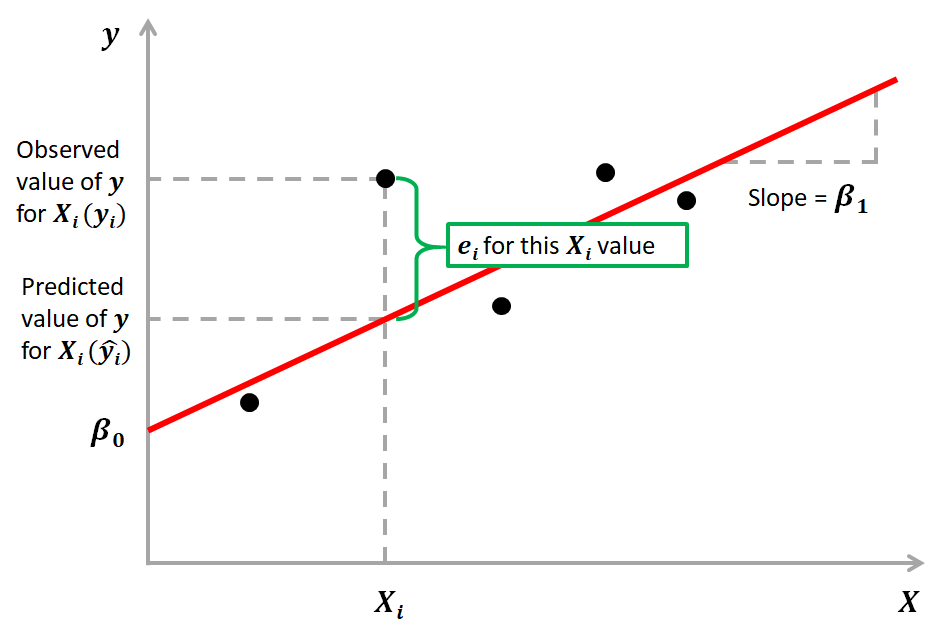How do we estimate β0 and β1? We can use a method called Ordinary Least Squares (OLS). The goal behind this is to minimize the distance from the black dots to the red line as close to zero as possible, which is done by minimizing the squared differences between actual and predicted outcomes. 我们如何估计β0   和β1 ？ 我们可以使用一种称为普通最小二乘(OLS)的方法 。   其背后的目标是使黑点到红线的距离尽可能地接近零，这是通过最小化实际结果与预测结果之间的平方差来实现的。
The difference between actual and predicted values is called residual (e) and can be negative or positive depending on whether the model overpredicted or underpredicted the outcome. Hence, to calculate the net error, adding all the residuals directly can lead to the cancellations of terms and reduction of the net effect. To avoid this, we take the sum of squares of these error terms, which is called the Residual Sum of Squares (RSS). 实际值与预测值之差称为残差(e)   可以是负值或正值，具体取决于模型是高估还是低估了结果。 因此，为了计算净误差，直接将所有残差相加会导致项的抵消和净效应的减小。 为了避免这种情况，我们采用这些误差项的平方和，称为残差平方和(RSS)。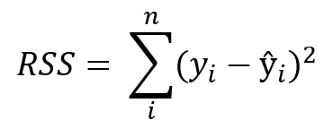The Ordinary Least Squares (OLS) method minimizes the residual sum of squares, and its objective is to fit a regression line that would minimize the distance (measured in quadratic values) from the observed values to the predicted ones (the regression line). 普通最小二乘法(OLS)方法使残差平方和最小化 ，其目的是拟合一条回归线，以使从观测值到预测值的距离(以二次值度量)最小化(回归线)。
多元线性回归(MLR) (Multiple Linear Regression (MLR))
Is the form of Linear Regression used when there are two or more predictors or input variables. Similar to the SLR model described before, it includes additional predictors: 是形式   有两个或多个预测变量或输入变量时使用的线性回归系数。 与之前描述的SLR模型类似，它包含其他预测变量：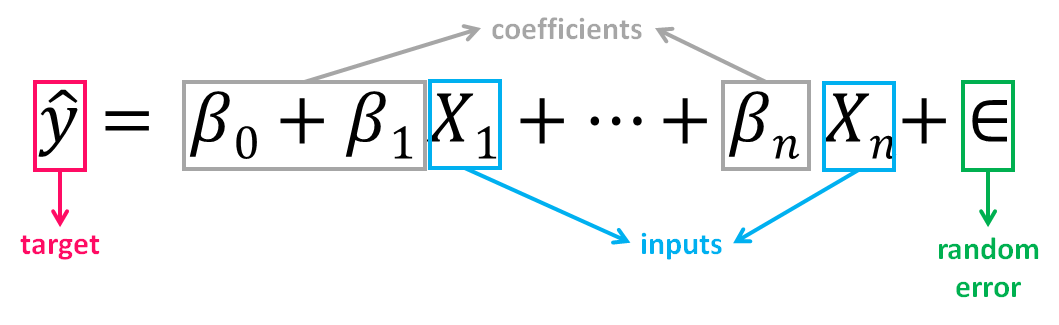Notice that the equation is just an extension of the Simple Linear Regression one, in which each input/ predictor has its corresponding slope coefficient (β). The first β term (β0) is the intercept constant and is the value of y in absence of all predictors (i.e when all X terms are 0). 注意，该方程只是简单线性回归方程的扩展，其中每个输入/预测变量都有其对应的斜率系数(β) 。 第一个β   项(β0)是截距常数，是在没有所有预测变量的情况下(即，当所有X项均为0时)的y值。
As the number of features grows, the complexity of our model increases and it becomes more difficult to visualize, or even comprehend, our data. Because there are more parameters in these models compared to SLR ones, more care is needed when working with them. Adding more terms will inherently improve the fit to the data, but the new terms may not have any real significance. This is dangerous because it can lead to a model that fits that data but doesn’t actually mean anything useful. 随着功能部件数量的增加，我们模型的复杂性也随之增加，并且更加难以可视化甚至理解我们的数据。 由于与SLR相比，这些模型中的参数更多，因此在使用它们时需要格外小心。 添加更多术语会从本质上改善数据的拟合度，但是新术语可能没有任何实际意义。 这很危险，因为它可能会导致模型适合该数据，但实际上并不意味着有用。
一个例子 (An example)
The advertising dataset consists of the sales of a product in 200 different markets, along with advertising budgets for three different media: TV, radio, and newspaper. We’ll use the dataset to predict the amount of sales (dependent variable), based on the TV, radio and newspaper advertising budgets (independent variables). 广告数据集包括产品在200个不同市场中的销售以及三种不同媒体(电视，广播和报纸)的广告预算。 我们将使用数据集根据电视，广播和报纸的广告预算(自变量)来预测销售量(自变量)。
Mathematically, the formula we’ll try solve is: 在数学上，我们将尝试解决的公式是：Finding the values of these constants (β) is what regression model does by minimizing the error function and fitting the best line or hyperplane (depending on the number of input variables). Let’s code. 通过最小化误差函数并拟合最佳直线或超平面(取决于输入变量的数量) ，回归模型可以找到这些常数(β)的值。 让我们编码。
加载数据并描述数据集 (Load data and describe dataset)
import pandas as pdimport numpy as npimport seaborn as snsimport matplotlib.pyplot as pltfrom sklearn.model_selection import train_test_splitfrom sklearn.linear_model import LinearRegressionfrom sklearn import metricsfrom sklearn.metrics import r2_scoreimport statsmodels.api as smNow we load the dataset: 现在我们加载数据集：
df = pd.read_csv(“Advertising.csv”)Let’s understand the dataset and describe it: 让我们了解数据集并对其进行描述：
df.head()We’ll drop the first column (“Unnamed”) since we don’t need it: 我们将删除第一列(“未命名”)，因为我们不需要它：
df = df.drop([‘Unnamed: 0’], axis=1)df.info()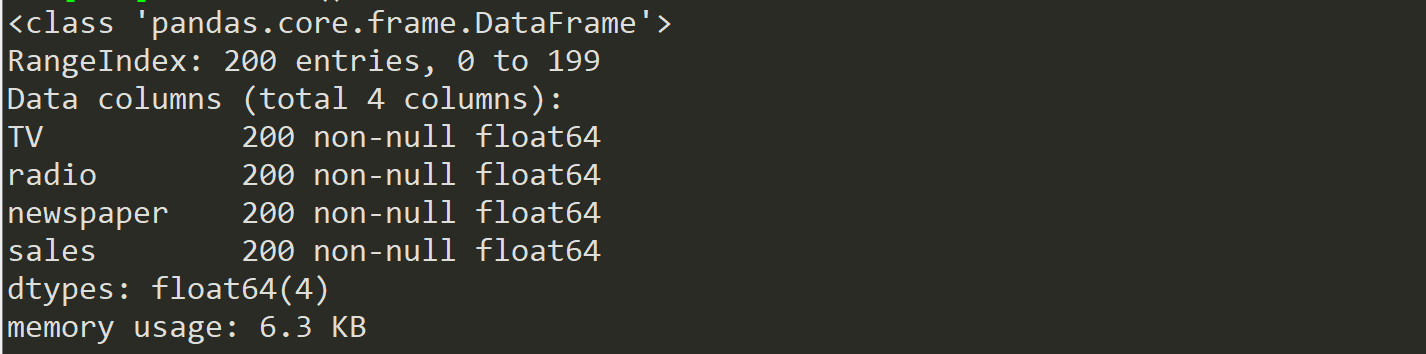Our dataset now contains 4 columns (including the target variable “sales”), 200 registers and no missing values. Let’s visualize the relationship between the independent and target variables. 现在，我们的数据集包含4列(包括目标变量“ sales”)，200个寄存器，并且没有缺失值。 让我们可视化自变量和目标变量之间的关系。
sns.pairplot(df)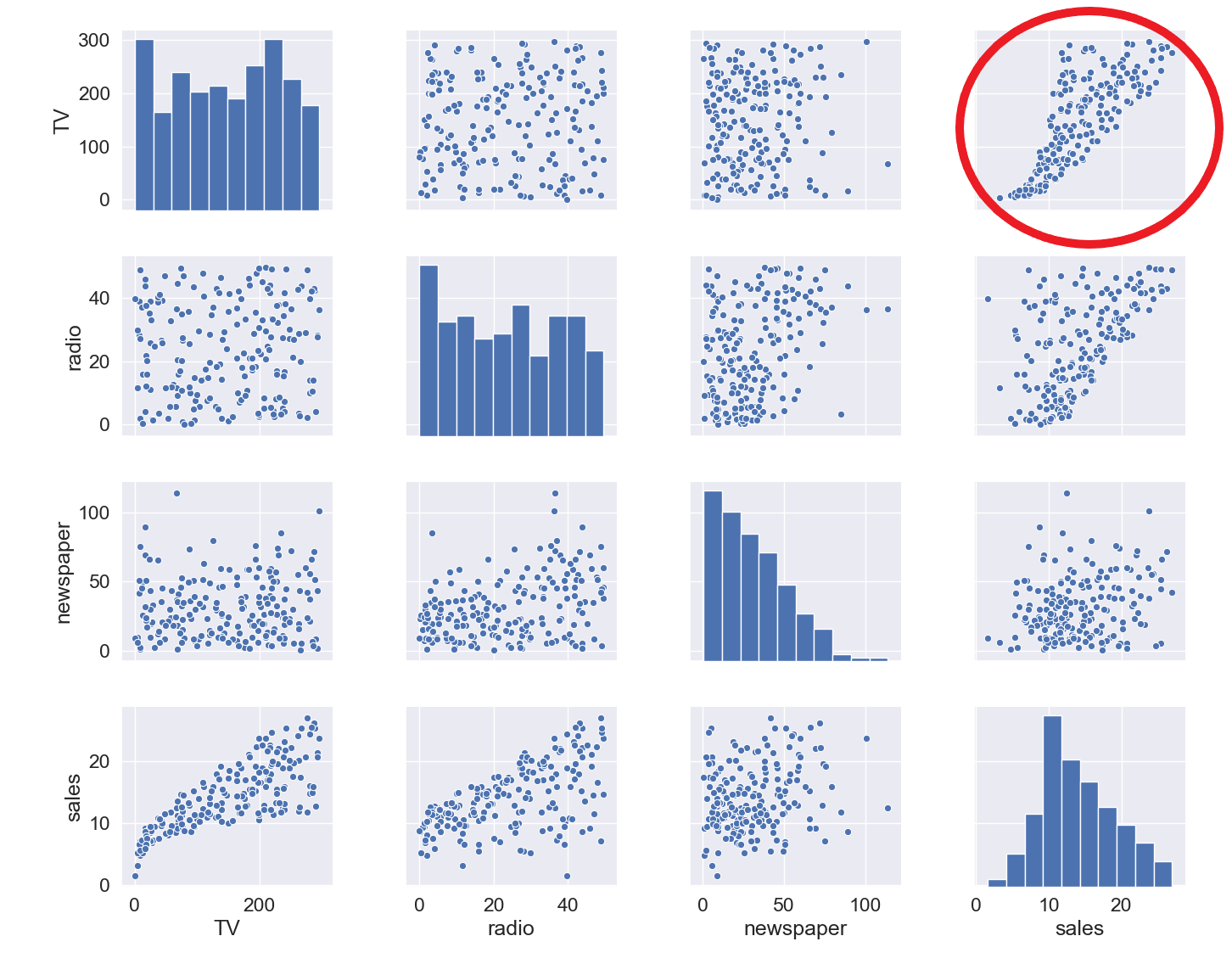The relationship between TV and sales seems to be pretty strong, and while there seems to be some trend between radio and sales, the relationship between newspaper and sales seems to be nonexistent. We can verify that also numerically through a correlation map: 电视与销售之间的关系似乎很牢固，虽然广播与销售之间似乎存在某种趋势，但报纸与销售之间的关系似乎不存在。 我们也可以通过相关图来数值验证：
mask = np.tril(df.corr())sns.heatmap(df.corr(), fmt=’.1g’, annot=True, cmap= ‘cool’, mask=mask)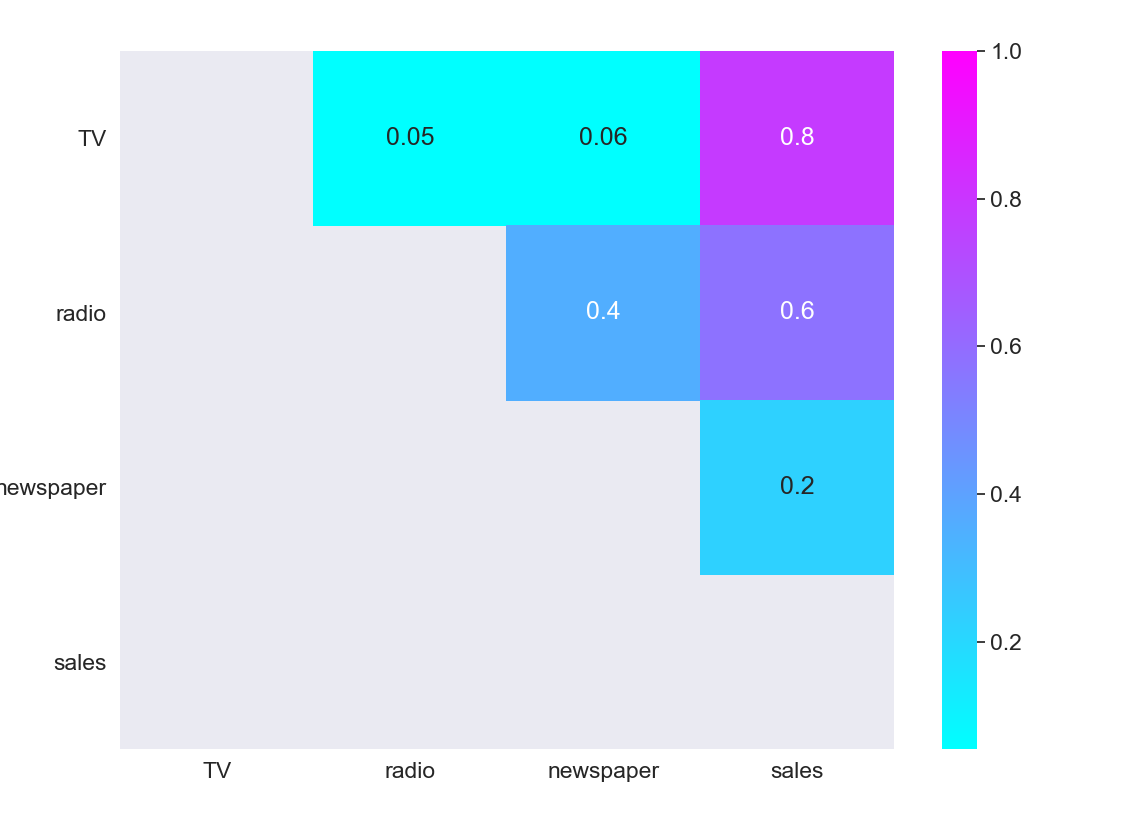As we expected, the strongest positive correlation happens between sales and TV, while the relationship between sales and newspaper is close to 0. 正如我们预期的那样，最强的正相关关系发生在销售和电视之间，而销售和报纸之间的关系接近于0。
选择特征和目标变量 (Select features and target variable)
Next, we divide the variables into two sets: dependent (or target variable “y”) and independents (or feature variables “X”) 接下来，我们将变量分为两组：因变量(或目标变量“ y”)和独立变量(或特征变量“ X”)
X = df.drop([‘sales’], axis=1)y = df[‘sales’] 分割数据集 (Split the dataset)
To understand model performance, dividing the dataset into a training set and a test set is a good strategy. By splitting the dataset into two separate sets, we can train using one set and test the model performance using unseen data on the other one. 为了了解模型的性能，将数据集分为训练集和测试集是一个很好的策略。 通过将数据集分为两个单独的集合，我们可以使用一个集合进行训练，而使用另一集合上的看不见的数据来测试模型的性能。
X_train, X_test, y_train, y_test = train_test_split(X, y, test_size=0.3, random_state=0)We split our dataset into 70% train and 30% test. The random_state parameter is used for initializing the internal random number generator, which will decide the splitting of data into train and test indices in your case. I set random state = 0 so that you can compare your output over multiple runs of the code using the same parameter. 我们将数据集分为70％训练和30％测试。 random_state参数用于初始化内部随机数生成器，它将根据您的情况决定将数据拆分为训练索引和测试索引。 我将随机状态设置为0，以便您可以使用同一参数在多个代码运行中比较输出。
print(X_train.shape,y_train.shape,X_test.shape,y_test.shape)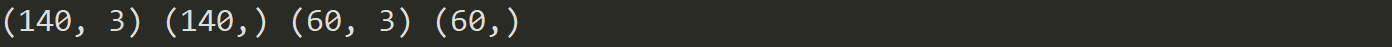By printing the shape of the splitted sets, we see that we created: 通过打印分割集的形状，我们看到我们创建了：
2 datasets of 140 registers each (70% of total registers), one with 3 independent variables and one with just the target variable, that will be used for training and producing the linear regression model. 2个数据集，每个140个寄存器(占总寄存器的70％)，一个包含3个独立变量，一个仅包含目标变量的数据集，将用于训练和生成线性回归模型。 2 datasets of 60 registers each (30% of total registers), one with 3 independent variables and one with just the target variable, that will be used for testing the performance of the linear regression model. 2个数据集，每个60个寄存器(占总寄存器的30％)，一个具有3个独立变量的数据集，一个仅具有目标变量的数据集，这些数据集将用于测试线性回归模型的性能。  建立模型 (Build model)
Building the model is as simple as: 建立模型非常简单：
mlr = LinearRegression() 火车模型 (Train model)
Fitting your model to the training data represents the training part of the modelling process. After it is trained, the model can be used to make predictions, with a predict method call: 使模型适合训练数据代表了建模过程中的训练部分。 训练后，可以使用预测方法调用该模型来进行预测：
mlr.fit(X_train, y_train)Let’s see the output of the model after being trained, and take a look at the value of β0 (the intercept): 让我们看一下训练后模型的输出，并看一下β0的值(截距)：
mlr.intercept_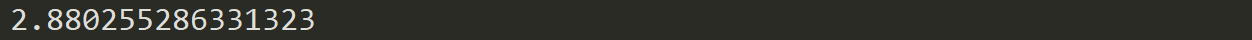We can also print the values of the coefficients (β): 我们还可以打印系数(β)的值：
coeff_df = pd.DataFrame(mlr.coef_, X.columns, columns =[‘Coefficient’])coeff_dfThis way we can now estimate the value of “sales” based on different budget values for TV, radio and newspaper: 这样，我们现在可以根据电视，广播和报纸的不同预算值来估算“销售”的价值：For example, if we determine a budget value of 50 for TV, 30 for radio and 10 for newspaper, the estimated value of “sales” will be: 例如，如果我们确定电视的预算值为50，广播的预算值为30，报纸的预算值为10，则“销售”的估算值将为：
example = [50, 30, 10]output = mlr.intercept_ + sum(example*mlr.coef_)output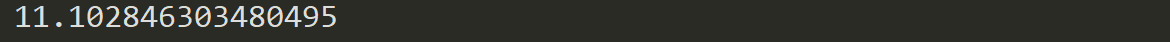测试模型 (Test model)
A test dataset is a dataset that is independent of the training dataset. This test dataset is the unseen data set for your model which will help you have a better view of its ability to generalize: 测试数据集是独立于训练数据集的数据集。 该测试数据集是您模型的看不见的数据集，有助于您更好地了解其概括能力：
y_pred = mlr.predict(X_test) 评估表现 (Evaluate Performance)
The quality of a model is related to how well its predictions match up against the actual values of the testing dataset: 模型的质量与预测与测试数据集的实际值的匹配程度有关：
print(‘Mean Absolute Error:’, metrics.mean_absolute_error(y_test, y_pred))print(‘Mean Squared Error:’, metrics.mean_squared_error(y_test, y_pred))print(‘Root Mean Squared Error:’, np.sqrt(metrics.mean_squared_error(y_test, y_pred)))print(‘R Squared Score is:’, r2_score(y_test, y_pred))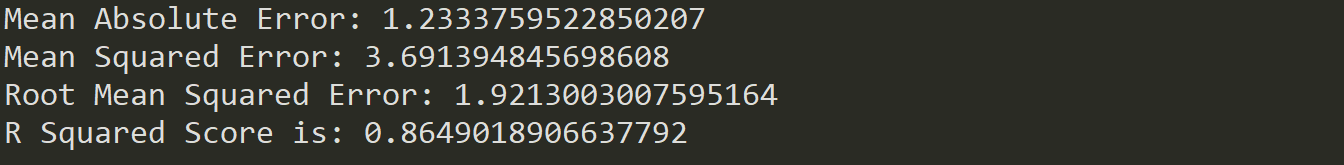After validating our model against the testing set, we get an R² of 0.86 which seems like a pretty decent performance score. But although a higher R² indicates a better fit for the model, it’s not always the case that a high measure is a positive thing. We’ll see below some ways to interpret and improve our regression models. 在根据测试集验证我们的模型后，我们得到的R²为0.86，这似乎是相当不错的性能得分。 但是，尽管较高的R²表示更适合该模型，但并非总是如此。 我们将在下面看到一些解释和改进回归模型的方法。
如何解释和改善您的模型？ (How to interpret and improve your model?)
OK, we created our model, and now what? Let’s take a look at the model statistics over the training data to get some answers: 好的，我们创建了模型，现在呢？ 让我们看一下训练数据上的模型统计信息，以获得一些答案：
X2 = sm.add_constant(X_train)model_stats = sm.OLS(y_train.values.reshape(-1,1), X2).fit()model_stats.summary()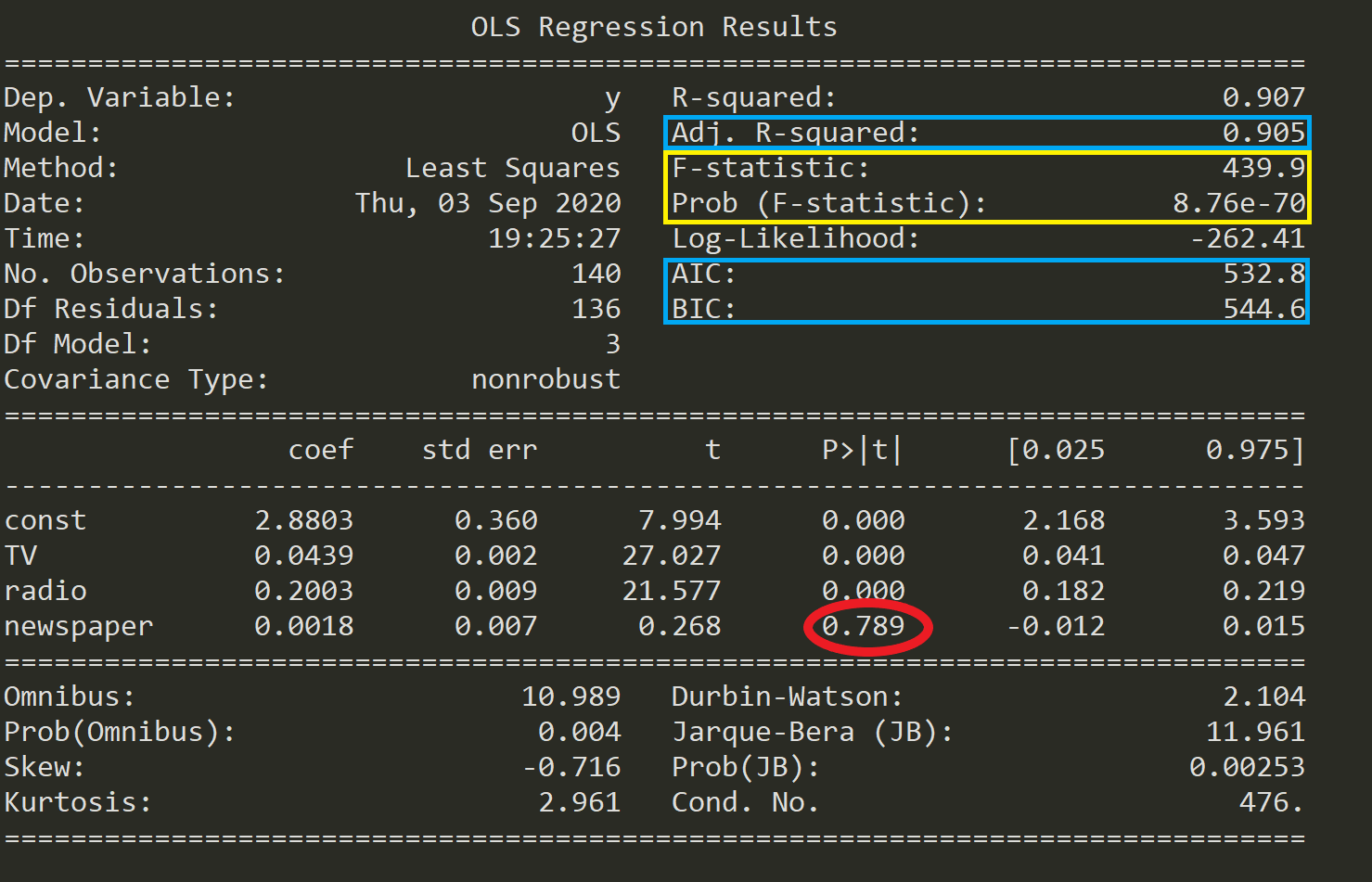Let’s see below what these numbers mean. 下面让我们看看这些数字的含义。
假设检验 (Hypothesis Test)
One of the fundamental questions you should answer while running a MLR model is, whether or not, at least one of the predictors is useful in predicting the output. What if the relationship between the independent variables and target is just by chance and there is no actual impact on sales due to any of the predictors? 运行MLR模型时，您应该回答的基本问题之一是， 至少有一个预测变量对预测输出有用 。 如果自变量与目标之间的关系仅仅是偶然的，并且由于任何预测因素而对销售没有实际影响，该怎么办？
We need to perform a Hypothesis Test to answer this question and check our assumptions. It all starts by forming a Null Hypothesis (H0), which states that all the coefficients are equal to zero, and there’s no relationship between predictors and target (meaning that a model with no independent variables fits the data as well as your model): 我们需要执行假设检验来回答这个问题并检查我们的假设。 这一切都始于形成一个零假设(H0) ，该假设指出所有系数都等于零，并且预测变量和目标变量之间没有关系(这意味着没有自变量的模型既适合数据又适合您的模型)：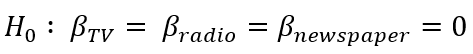Towards Data Science迈向数据科学 On the other hand, we need to define an Alternative Hypothesis (Ha), which states that at least one of the coefficients is not zero, and there is a relationship between predictors and target (meaning that your model fits the data better than the intercept-only model): 另一方面，我们需要定义一个替代假设(Ha) ，该假设指出至少一个系数不为零，并且预测变量与目标之间存在关系(这意味着您的模型比截距更适合数据仅限型号)：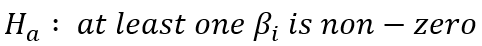Towards Data Science迈向数据科学 If we want to reject the Null Hypothesis and have confidence in our regression model, we need to find strong statistical evidence. To do this we perform a hypothesis test, for which we use the F-Statistic. 如果我们想拒绝零假设并对我们的回归模型有信心，我们需要找到有力的统计证据。 为此，我们执行假设检验，为此我们使用F统计量 。
If the value of F-statistic is equal to or very close to 1, then the results are in favor of the Null Hypothesis and we fail to reject it. 如果F统计量的值等于或非常接近1，则结果支持零假设，我们无法拒绝它。 As we can see in the table above (marked in yellow), the F-statistic is 439.9, thus providing strong evidence against the Null Hypothesis (that all coefficients are zero). Next, we also need to check the probability of occurrence of the F-statistic (also marked in yellow) under the assumption that the null hypothesis is true, which is 8.76e-70, an exceedingly small number lower than 1%. This means that there is much less than 1% probability that the F-statistic of 439.9 could have occurred by chance under the assumption of a valid Null hypothesis. 正如我们在上表中看到的(以黄色标记)，F统计量为439.9，因此有力地证明了零假设(所有系数均为零)。 接下来，我们还需要在原假设为真(8.76e-70)的情况下，检查F统计量 (也用黄色标记) 的发生概率 ，该数值非常小，低于1％。 这意味着在有效的Null假设的假设下，偶然发生F统计量439.9的可能性要小于1％。
Having said this, we can reject the Null Hypothesis and be confident that at least one predictor is useful in predicting the output. 话虽如此，我们可以否定零假设，并相信至少有一个预测变量可用于预测输出。
产生模型 (Generate models)
Running a Linear Regression model with many variables including irrelevant ones will lead to a needlessly complex model. Which of the predictors are important? Are all of them significant to our model? To find that out, we need to perform a process called feature selection. The 2 main methods for feature selection are: 运行包含许多不相关变量的线性回归模型将导致不必要的复杂模型。 哪些预测指标很重要？ 它们对我们的模型都重要吗？ 为了找出答案，我们需要执行一个称为功能选择的过程。   特征选择的2种主要方法是：
Forward Selection: where predictors are added one at a time beginning with the predictor with the highest correlation with the dependent variable. Then, variables of greater theoretical importance are incorporated to the model sequentially, until a stopping rule is reached. 正向选择：从与因变量相关性最高的预测变量开始，一次将预测变量添加一次。 然后，将具有较大理论重要性的变量顺序合并到模型中，直到达到停止规则为止。 Backward Elimination: where you start with all variables in the model, and remove the variables that have the least statistically significant (greater p-value), until a stopping rule is reached. 向后消除：从模型中的所有变量开始，然后删除统计上意义最小的变量(较大的p值)，直到达到停止规则为止。 Although both methods can be used, unless the number of predictors is larger than the sample size (or number of events), it’s usually preferred to use a backward elimination approach. 尽管两种方法都可以使用，但是除非预测变量的数量大于样本大小(或事件的数量)，否则通常首选使用向后消除方法。
You can find a full example and implementation of these methods in this link. 您可以在此链接中找到这些方法的完整示例和实现。
比较型号 (Compare models)
Every time you add an independent variable to a model, the R² increases, even if the independent variable is insignificant. In our model, are all predictors contributing to an increase in sales? And if so, are they all doing it in the same extent? 每当您向模型添加自变量时，即使自变量无关紧要，R²也会增加。 在我们的模型中，所有预测因素是否都有助于增加销售额？ 如果是这样，他们是否都在同一程度上做到这一点？
As opposed to R², Adjusted R² is a measure that increases only when the independent variable is significant and affects the dependent variable. So,if your R² score increases but the Adjusted R² score decreases as you add variables to the model, then you know that some features are not useful and you should remove them. 与R²相对，“ 调整R²”是仅在自变量显着且影响因变量时才增加的量度。 因此，如果在向模型中添加变量时R²分数增加而AdjustedR²分数减少，则说明某些功能没有用，应将其删除。
An interesting finding in the table above is that the p-value for newspaper is super high (0.789, marked in red). Finding the p-value for each coefficient will tell if the variable is statistically significant to predict the target or not. 上表中的一个有趣发现是报纸的p值超高(0.789，红色标记)。 查找每个系数的p值将说明该变量在统计上是否对预测目标有意义。
As a general rule of thumb, if the p-value for a given variable is less than 0.05 then there is a strong relationship between that variable and the target. 作为一般经验法则，如果给定变量的p值小于0.05，则该变量与目标之间存在很强的关系。 This way, including the variable newspaper doesn’t seem to be appropriate to reach a robust model, and removing it may improve the performance and generalization of the model. 这样，包括可变报纸似乎并不适合建立一个健壮的模型，删除它可以改善模型的性能和通用性。
Besides Adjusted R² score you can use other criteria to compare different regression models: 除了调整后的R²得分外，您还可以使用其他条件比较不同的回归模型：
Akaike Information Criterion (AIC): is a technique used to estimate the likelihood of a model to predict/estimate the future values. It rewards models that achieve a high goodness-of-fit score and penalizes them if they become overly complex. A good model is the one that has minimum AIC among all the other models. 赤池信息准则(AIC)：是一种用于估计模型预测/估计未来价值的可能性的技术。 它会奖励获得高拟合优度分数的模型，如果模型过于复杂，则会对其进行惩罚。 一个好的模型是所有其他模型中具有最小AIC的模型。 Bayesian Information Criterion (BIC): is another criteria for model selection that measures the trade-off between model fit and complexity, penalizing overly complex models even more than AIC. 贝叶斯信息准则(BIC)：是模型选择的另一个标准，它衡量模型拟合与复杂度之间的权衡，对过于复杂的模型造成的惩罚甚至超过了AIC。  假设条件 (Assumptions)
Because Linear Regression models are an approximation of the long-term sequence of any event, they require some assumptions to be made about the data they represent in order to remain appropriate. Most statistical tests rely upon certain assumptions about the variables used in the analysis, and when these assumptions are not met, the results may not be trustworthy (e.g. resulting in Type I or Type II errors). 由于线性回归模型是任何事件的长期序列的近似值，因此需要对它们表示的数据进行一些假设才能保持适当。 大多数统计检验都依赖于有关分析中使用的变量的某些假设，如果不满足这些假设，则结果可能不可信(例如，导致I型或II型错误)。
Linear Regression models are linear in the sense that the output is a linear combination of the input variables, and only suited for modeling linearly separable data. Linear Regression models work under various assumptions that must be present in order to produce a proper estimation and not to depend solely on accuracy scores: 从输出是输入变量的线性组合的意义上讲，线性回归模型是线性的，并且仅适用于对线性可分离数据进行建模。 线性回归模型在各种假设下工作，这些假设必须存在才能产生适当的估计，而不仅仅是依赖于准确性得分：
Linearity: the relationship between the features and target must be linear. One way to check the linear relationships is to visually inspect scatter plots for linearity. If the relationship displayed in the scatter plot is not linear, then we’d need to run a non-linear regression or transform the data. 线性 ：特征与目标之间的关系必须是线性的。 检查线性关系的一种方法是目视检查散点图的线性。 如果散点图中显示的关系不是线性的，那么我们需要运行非线性回归或转换数据。 Homoscedasticity: the variance of the residual must be the same for any value of x. Multiple linear regression assumes that the amount of error in the residuals is similar at each point of the linear model. This scenario is known as homoscedasticity. Scatter plots are a good way to check whether the data are homoscedastic, and also several tests exist to validate the assumption numerically (e.g. Goldfeld-Quandt, Breusch-Pagan, White) 均方差：对于任何x值，残差的方差必须相同。 多元线性回归假设残差的误差量在线性模型的每个点都相似。 这种情况称为同调。 散点图是检查数据是否为同方差的好方法，并且还存在一些测试以数值验证该假设(例如，Goldfeld-Quandt，Breusch-Pagan，White)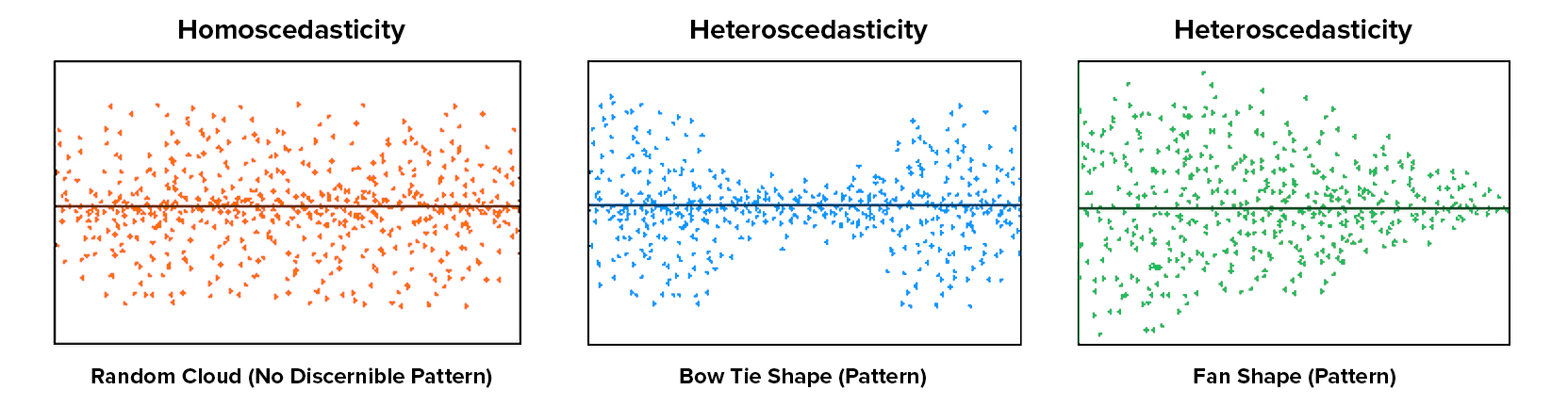Towards Data Science迈向数据科学 No multicollinearity: data should not show multicollinearity, which occurs when the independent variables (explanatory variables) are highly correlated to one another. If this happens, there will be problems in figuring out the specific variable that contributes to the variance in the dependent/target variable. This assumption can be tested with the Variance Inflation Factor (VIF) method, or through a correlation matrix. Alternatives to solve this issue may be centering the data (deducting the mean score), or conducting a factor analysis and rotating the factors to insure independence of the factors in the linear regression analysis. 无多重共线性：数据不应显示多重共线性，当自变量(解释变量)彼此高度相关时，就会发生多重共线性。 如果发生这种情况，将很难找出导致因变量/目标变量差异的特定变量。 可以使用方差通货膨胀系数(VIF)方法或通过相关矩阵来检验此假设。 解决此问题的替代方法可能是将数据居中(扣除平均得分)，或进行因子分析并旋转因子以确保线性回归分析中因子的独立性。 No autocorrelation: the value of the residuals should be independent of one another. The presence of correlation in residuals drastically reduces model’s accuracy. If the error terms are correlated, the estimated standard errors tend to underestimate the true standard error. To test for this assumption, you can use the Durbin-Watson statistic. 无自相关 ：残差的值应彼此独立。 残差中存在相关性会大大降低模型的准确性。 如果误差项相关，则估计的标准误差往往会低估真实的标准误差。 要测试此假设，可以使用Durbin-Watson统计信息。 Normality of residuals: residuals must be normally distributed. Normality can be checked with a goodness of fit test (e.g. Kolmogorov-Smirnov or Shapiro-Wilk tests), and if data is not normally distributed, a non-linear transformation (e.g. log transformation) might fix the issue. 残差的正态性 ：残差必须正态分布。 可以使用拟合优度检验(例如Kolmogorov-Smirnov或Shapiro-Wilk检验)来检查正态性，如果数据不是正态分布的，则非线性转换(例如对数转换)可以解决此问题。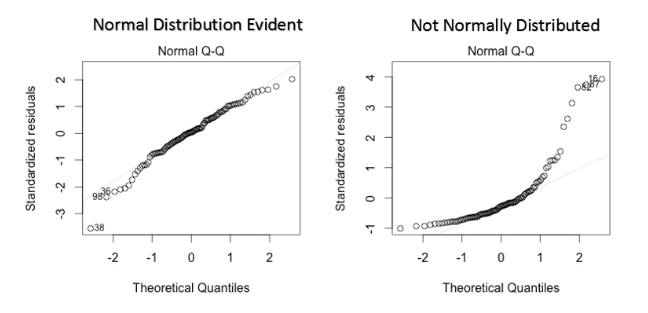Towards Data Science迈向数据科学 Assumptions are critical because if they are not valid, then the analytical process can be considered unreliable, unpredictable, and out of control. Failing to meet the assumptions can lead to draw conclusions that are not valid or scientifically unsupported by the data. 假设是至关重要的，因为如果假设无效，那么分析过程将被认为是不可靠，不可预测且不受控制的。 不符合这些假设可能导致得出无效的结论或数据在科学上不支持的结论。
You can find a full testing of the assumptions in this link. 您可以在此链接中找到对假设的完整测试。
最后的想法 (Final thoughts)
Although MLR models extend the scope of SLR models, they are still linear models, meaning that the terms included in the model are incapable of showing any non-linear relationships between each other or representing any sort of non-linear trend. You should also be careful when predicting a point outside the observed range of features since the relationship among variables may change as you move outside the observed range (a fact that you can’t know because you don’t have the data). 尽管MLR模型扩展了SLR模型的范围，但它们仍然是线性模型，这意味着模型中包含的术语无法显示彼此之间的任何非线性关系或表示任何种类的非线性趋势。 在预测要素的观察范围之外的点时，您还应该小心，因为变量之间的关系可能会随着您移出观察范围而改变(这是您不知道的原因，因为您没有数据)。
The observed relationship may be locally linear, but there may be unobserved non-linear relationships on the outside range of your data. 观察到的关系可能是局部线性的，但是在数据的外部范围上可能存在未观察到的非线性关系。 Linear models can also model curvatures by including non-linear variables such as polynomials and transforming exponential functions. The linear regression equation is linear in the parameters, meaning you can raise an independent variable by an exponent to fit a curve, and still remain in the “linear world”. Linear Regression models can contain log terms and inverse terms to follow different kinds of curves and yet continue to be linear in the parameters. 线性模型还可以通过包含非线性变量(例如多项式)和变换指数函数来对曲率建模 。 线性回归方程的 参数是线性的 ，这意味着您可以通过指数增加自变量以拟合曲线，但仍保留在“线性世界”中。 线性回归模型可以包含对数项和逆项，以遵循不同类型的曲线，但参数仍保持线性。While the independent variable is squared, the model is still linear in the parametersRegressions like Polynomial Regression can model non-linear relationships, and while a linear equation has one basic form, non-linear equations can take many different forms. The reason you might consider using Non-linear Regression Models is that, while linear regression can model curves, it might not be able to model the specific curve that exists in your data. 像多项式回归这样的回归可以对非线性关系进行建模，虽然线性方程式具有一种基本形式，但是非线性方程式可以采用许多不同形式。 您可能会考虑使用非线性回归模型的原因是，尽管线性回归可以对曲线进行建模，但它可能无法对数据中存在的特定曲线进行建模。
You should also know that OLS is not the only method to fit your Linear Regression model, and other optimization methods like Gradient Descent are more adequate to fit large datasets. Applying OLS to complex and non-linear algorithms might not be scalable, and Gradient Descent can be computationally cheaper (faster) for finding the solution. Gradient Descent is an algorithm that minimizes functions, and given a function defined by a set of parameters, the algorithm starts with an initial set of parameter values and iteratively moves toward a set of parameter values that minimize the function. This iterative minimization is achieved using derivatives, taking steps in the negative direction of the function gradient. 您还应该知道，OLS并不是拟合线性回归模型的唯一方法，而其他优化方法(如Gradient Descent)更适合于大型数据集。 将OLS应用于复杂和非线性算法可能无法扩展，并且Gradient Descent在计算上可能更便宜(更快)以找到解决方案。 梯度下降(Gradient Descent)是一种使函数最小化的算法 ，并且在给定由一组参数定义的函数的情况下，该算法从一组初始参数值开始，然后逐步向一组参数值最小化该函数。 使用导数可以在函数梯度的负方向上采取步骤来实现这种迭代最小化 。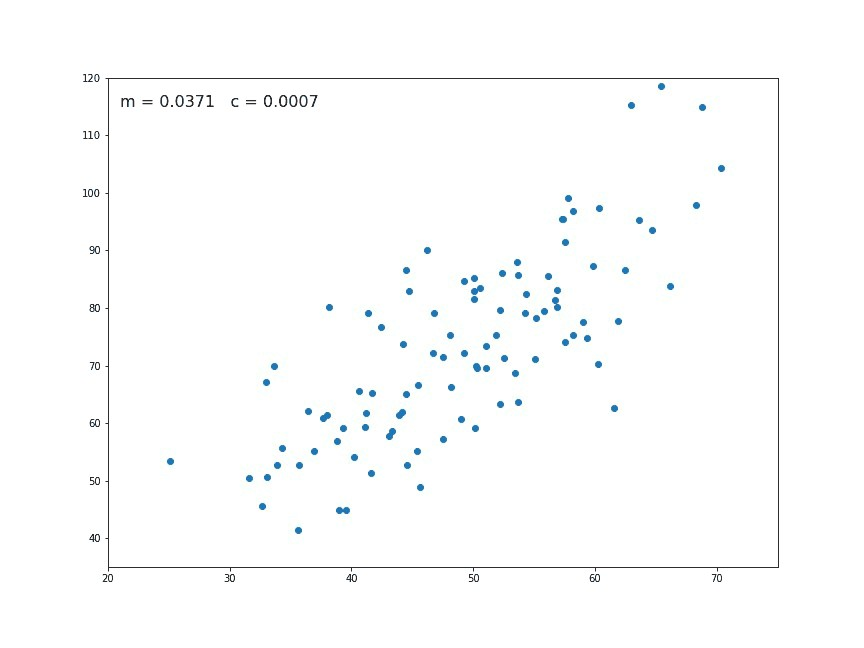Towards Data Science迈向数据科学 Another key thing to take into account is that outliers can have a dramatic effect on regression lines and the correlation coefficient. In order to identify them it’s essential to perform Exploratory Data Analysis (EDA), examining the data to detect unusual observations, since they can impact the results of our analysis and statistical modeling in a drastic way. In case you recognize any, outliers can be imputed (e.g. with mean / median / mode), capped (replacing those outside certain limits), or replaced by missing values and predicted. 要考虑的另一项关键是， 离群值会对回归线和相关系数产生巨大影响 。 为了识别它们，执行探索性数据分析(EDA) ，检查数据以检测异常观察非常重要，因为它们会以极大的方式影响我们的分析和统计建模的结果。 如果您识别出任何异常，则可以对异常值进行估算(例如，使用均值/中位数/众数)，设置上限(替换超出某些限制的那些值)或替换为缺失值并进行预测。
Finally, some limitations of Linear Regression models are: 最后， 线性回归模型的一些局限性是：
Omitted variables. It is necessary to have a good theoretical model to suggest variables that explain the dependent variable. In the case of a simple two-variable regression, one has to think of other factors that might explain the dependent variable, since there may be other “unobserved” variables that explain the output. 省略的变量 。 必须有一个良好的理论模型来提出解释因变量的变量。 在简单的二变量回归的情况下，必须考虑可能解释因变量的其他因素，因为可能会有其他“无法观察的”变量解释输出。 Reverse causality. Many theoretical models predict bidirectional causality — that is, a dependent variable can cause changes in one or more explanatory variables. For instance, higher earnings may enable people to invest more in their own education, which, in turn, raises their earnings. This complicates the way regressions should be estimated, calling for special techniques. 反向因果关系 。 许多理论模型都预测双向因果关系，即因变量可能导致一个或多个解释变量发生变化。 例如，更高的收入可能使人们能够对自己的教育进行更多的投资，从而增加了他们的收入。 这使估计回归的方式变得复杂，需要特殊的技术。 Mismeasurement. Factors might be measured incorrectly. For example, aptitude is difficult to measure, and there are well-known problems with IQ tests. As a result, the regression using IQ might not properly control for aptitude, leading to inaccurate or biased correlations between variables like education and earnings. 测量错误 。 可能会错误地评估因素。 例如，能力很难测量，并且智商测试存在众所周知的问题。 结果，使用智商的回归可能无法适当地控制才能，导致教育和收入等变量之间的关系不准确或有偏见。 Too limited a focus. A regression coefficient provides information only about how small changes — not large changes — in one variable relate to changes in another. It will show how a small change in education is likely to affect earnings but it will not allow the researcher to generalize about the effect of large changes. If everyone became college educated at the same time, a newly minted college graduate would be unlikely to earn a great deal more because the total supply of college graduates would have increased dramatically. 焦点太有限了 。 回归系数仅提供有关一个变量中的微小变化(而不是大变化)与另一变量中的变化之间的关系的信息。 它会显示出教育的微小变化可能会如何影响收入，但不会使研究人员对较大变化的影响进行概括。 如果每个人都同时接受大学教育，那么刚毕业的大学毕业生就不太可能赚更多的钱，因为大学毕业生的总供应量将大大增加。 Interested in these topics? Follow me on Linkedin or Twitter 对这些主题感兴趣？ 在Linkedin或Twitter上关注我 翻译自: https://towardsdatascience.com/your-guide-to-linear-regression-models-df1d847185db一般线性模型和线性回归模型
展开全文机器学习 python 人工智能 深度学习
• ## 多元线性回归模型

万次阅读 多人点赞 2019-07-02 19:22:12
多元线性回归模型通常用来研究一个应变量依赖多个自变量的变化关系，如果二者的以来关系可以用线性形式来刻画，则可以建立多元线性模型来进行分析。 1.模型简介 1.1模型的结构 多元线性回归模型通常用来描述变脸y和x...
多元线性回归模型通常用来研究一个应变量依赖多个自变量的变化关系，如果二者的以来关系可以用线性形式来刻画，则可以建立多元线性模型来进行分析。
1.模型简介
1.1模型的结构
多元线性回归模型通常用来描述变量y和x之间的随机线性关系，即：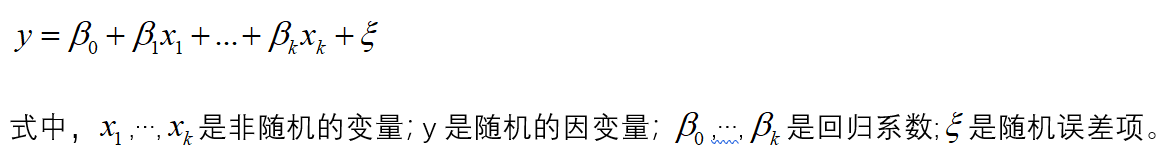如果对y和x进行了x次观测，得到n组观察值yi,x1i,…,xki(i=1,2,…,n),他们满足一下关系式：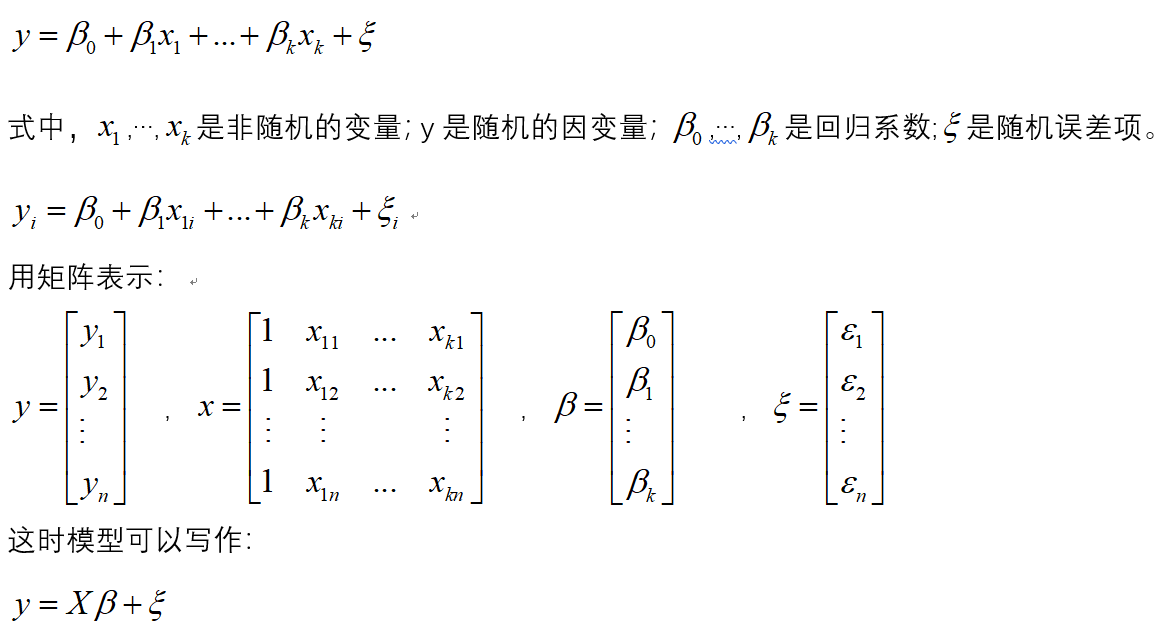1.2模型参数的检验
在正态假定下，如果X是列满秩的，则普通线性回归模型的参数最小二乘估计为：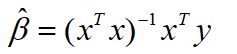于是y的估计值为：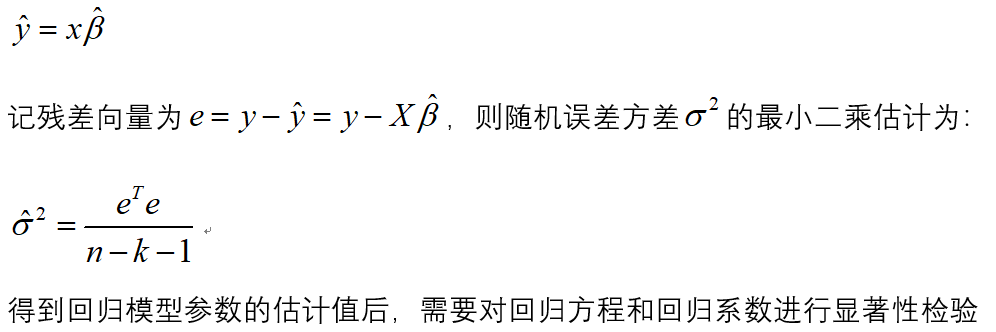（1）回归方程的显著性检验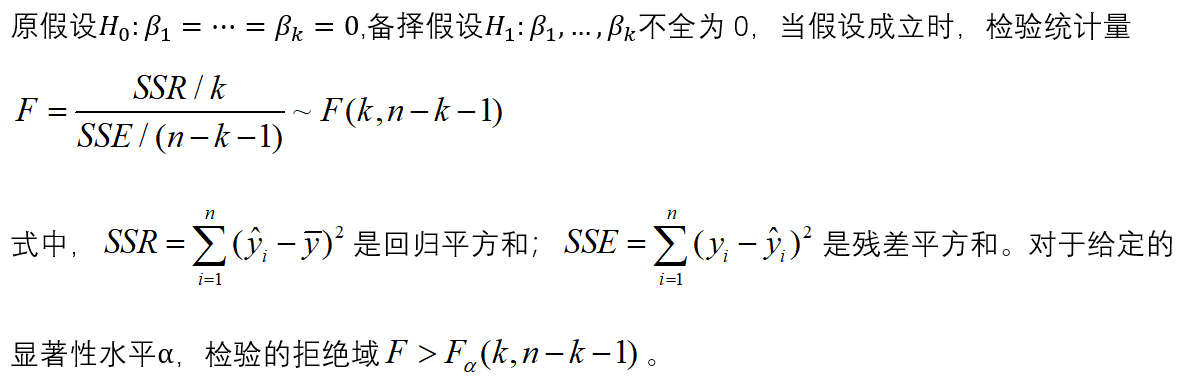（2）回归系数的显著性检验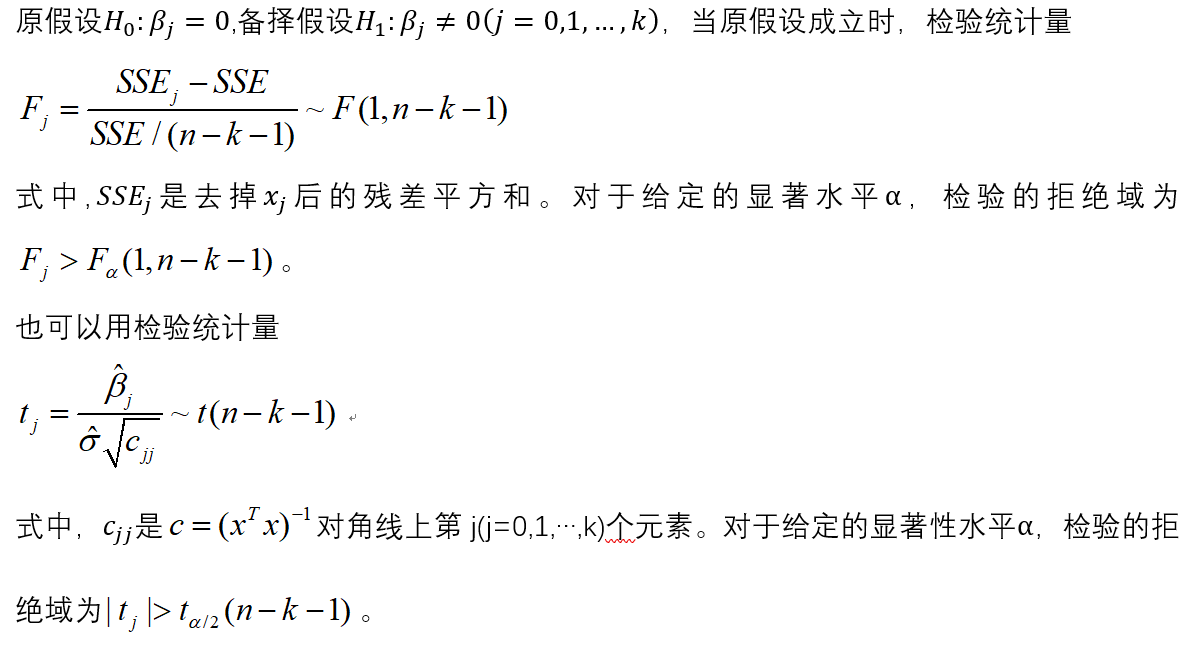2.建模步骤
（1）根据数据建立回归模型
（2）对模型进行显著性检验
（3)  对模型进行回归诊断
3.建模
library(car)
a
lm.salary=lm(锘縴~x1+x2+x3+x4,data=a)
summary(lm.salary)
#注：锘縴是y乱码之后的结果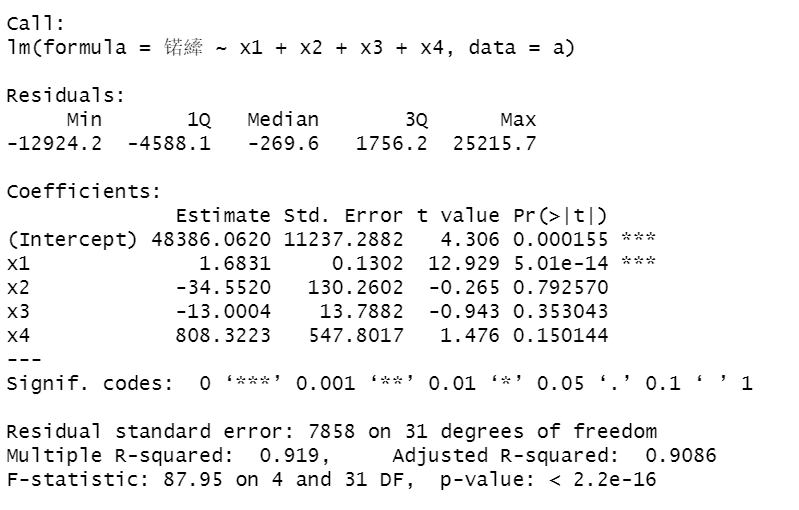发现x2,x3,x4系数不显著。
（2）对变量进行选择
lm.step=step(lm.salary,direction="both")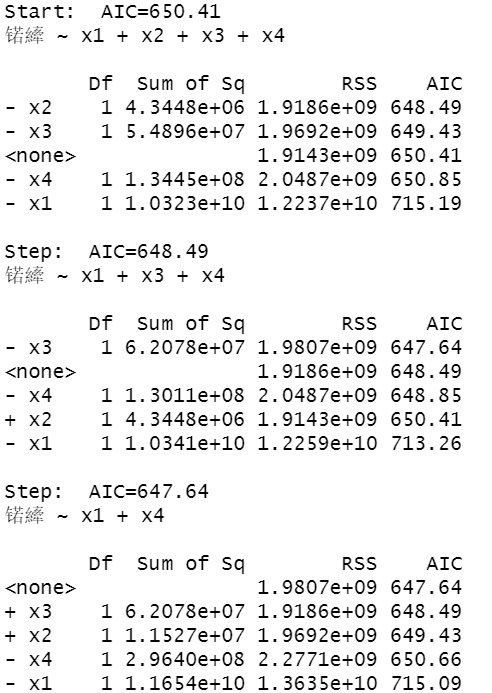如果去掉变量x2,AIC的值为648.49，如果去掉变量x3,AIC的值为650.85，如果去掉变量x1,AIC的值为715.19，所以在这里去掉x2.
进行下一轮的计算：
lm.salary=lm(锘縴~x1+x3+x4,data=a)
lm.step=step(lm.salary,direction="both")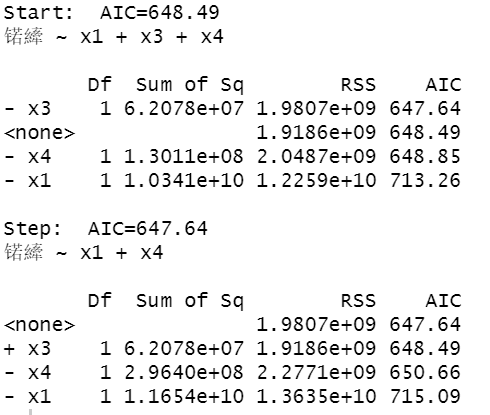发现去掉x3，AIC 的值为647.64，所以去掉x3.
单独对x1和x4，进行拟合。
lm.salary=lm(锘縴~x1+x4,data=a)
summary(lm.salary)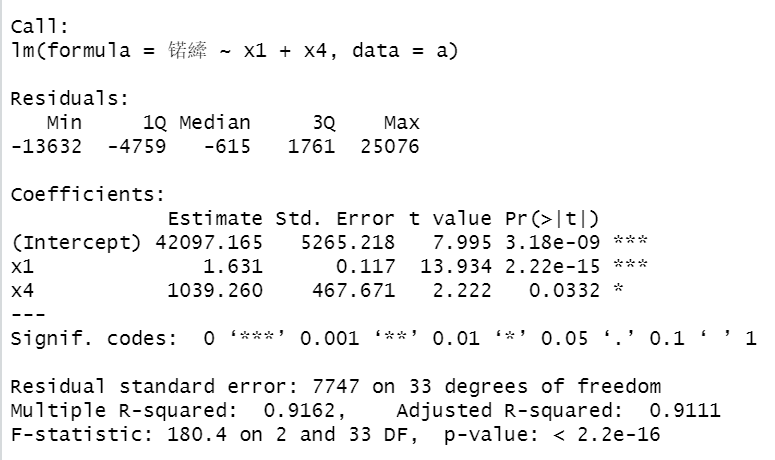可以看出F检验P值小于0.05显著，各个参数系数也是显著的。
（3）对上述回归模型进行回归残差诊断
算出模型的标准化残差
library(TSA)
y.rst=rstandard(lm.step)
y.rst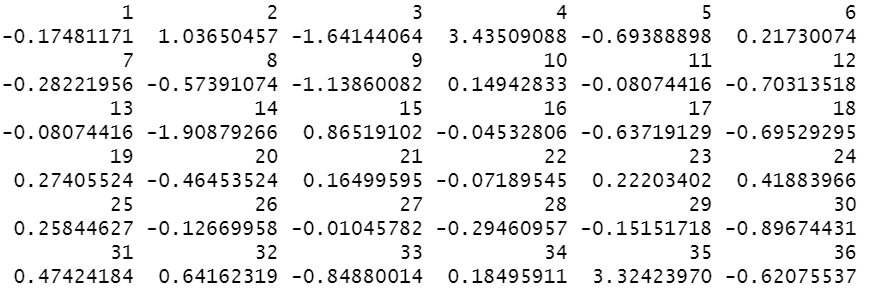画出其残差散点图：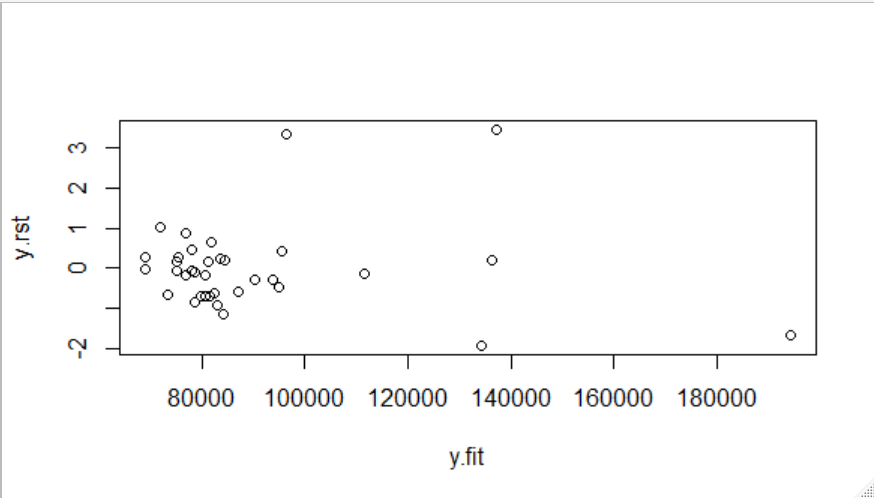很明显发现4和35号点异常，将这两个点去除。
lm.salary=lm(log(锘縴)~x1+x2+x3+x4,data=a[-c(4,35),])
lm.step=step(lm.salary,direction="both")
y.rst=rstandard(lm.step)
y.fit=predict(lm.step)
plot(y.rst~y.fit)

去除两点后的结果：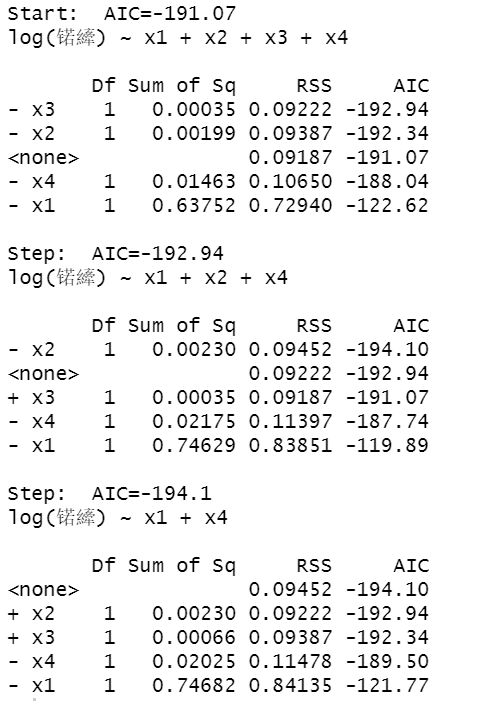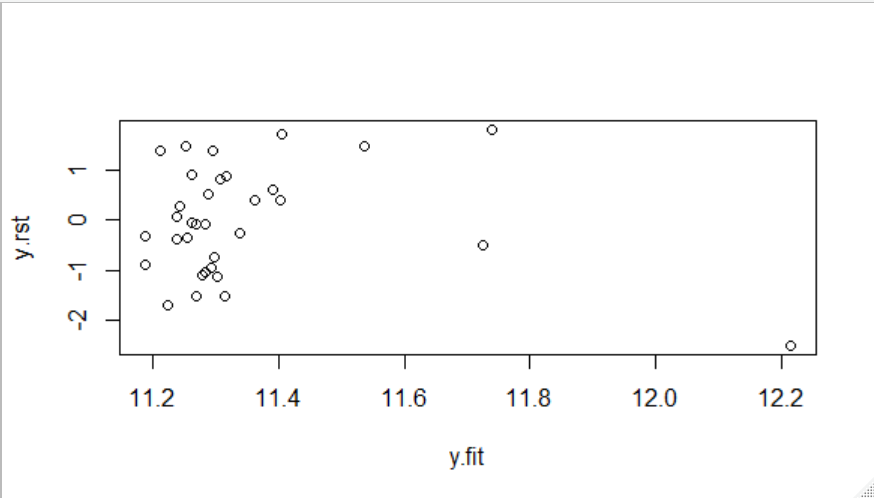绘制模型诊断图：
par(mfrow=c(2,2))
plot(lm.step)
influence.measures(lm.step)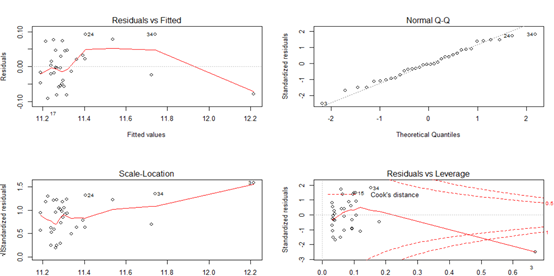残差拟合图基本上呈现随机分布模式，正态Q-Q图基本落在直线上，表明残差服从正态分布；大小-位置图和残差-杠杆图以小组的形式存在并且离中心不远。这说明3，4，35号观测值可能是异常点和强影响点。


展开全文• 线性回归模型，是利用数理统计中回归分析，来确定两种或两种以上变量间相互依赖的定量关系的一种统计分析方法，运用十分广泛。其表达形式为y = w'x+e，e为误差服从均值为0的正态分布。线性回归模型是利用称为线性...
线性就是每个变量的指数都是1，而非线性就是至少有一个变量的指数不是1。

通过指数来进行判断即可。
线性回归模型，是利用数理统计中回归分析，来确定两种或两种以上变量间相互依赖的定量关系的一种统计分析方法，运用十分广泛。其表达形式为y = w'x+e，e为误差服从均值为0的正态分布。线性回归模型是利用称为线性回归方程的最小平方函数对一个或多个自变量和因变量之间关系进行建模的一种回归分析。这种函数是一个或多个称为回归系数的模型参数的线性组合。只有一个自变量的情况称为简单回归,大于一个自变量情况的叫做多元回归。
非线性回归，是在掌握大量观察数据的基础上，利用数理统计方法建立因变量与自变量之间的回归关系函数表达式（称回归方程式）。回归分析中，当研究的因果关系只涉及因变量和一个自变量时，叫做一元回归分析；当研究的因果关系涉及因变量和两个或两个以上自变量时，叫做多元回归分析。
展开全文• 多元线性回归模型评估You’ve got a sample dataset and just finished working on a machine learning algorithm using the linear regression model. But now, you are wondering whether or not your analysis ...机器学习 python 人工智能 深度学习
• 线性回归模型请看上篇文章，本篇文章介绍的是非线性回归模型 线性回归模型链接 在目前的机器学习领域中，最常见的三种任务就是：回归分析、分类分析、聚类分析。那么什么是回归呢？回归分析是一种预测性的建模技术，...
• 线性回归模型(Linear Regression)及Python实现1.模型对于一份数据，它有两个变量，分别是Petal.Width和Sepal.Length，画出它们的散点图。我们希望可以构建一个函数去预测Sepal.Length，当我们输入Petal.Width时，...
• 线性回归模型是数据科学领域最简单的模型，很多复杂的模型 （如多项式回归、逻辑回归、SVM） 都是建立在线性回归模型的基础上的，或者是从其中能找到线性回归模型的影子。最简单的线性回归模型就是一元线性回归模型...损失函数 机器学习 最小二乘法
• sklearn实现非线性回归模型前言： sklearn实现非线性回归模型的本质是通过线性模型实现非线性模型，如何实现呢？sklearn就是先将非线性模型转换为线性模型，再利用线性模型的算法进行训练模型。一、线性模型解决非...
• （1）模型准备多元线性回归模型是指含有多个解释变量的线性回归模型，用于解释被解释的变量与其他多个变量解释变量之间的线性关系。其数学模型为： 上式表示一种 元线性回归模型，可以看出里面共有 个解释变量。表示...多元线性回归数据 多元线性回归数据集
• 我们可以使用线性回归模型来拟合数据，然而，在现实中，数据未必总是线性（或接近线性）的。当数据并非线性时，直接使用LinearRegression的效果可能会较差，产生欠拟合。 import numpy as np import matplotlib as...机器学习
• 为什么讲线性回归模型？1、线性回归模型是最基础的模型，我们之前讲了很多高大上的模型，却忽视了这个模型，因此想对这个模型有进一步了解；2、线性回归模型用起来很简单，但是看了很多资料后，发现它的原理并不也很...
• 1、线性回归模型 2. 销售收入预测 3. 导入数据 4. 处理日期数据 5. 建立线性回归模型，利用线性拟合函数进行预测python
• 文章目录一、具体实现步骤第1步：数据预处理第2步：使用简单线性回归模型来训练训练集第3步：预测结果第4步：可视化训练集结果可视化测试集结果可视化二、可视化结果展示训练集结果可视化结果测试集结果可视化结果三...机器学习
• 线性回归模型常用训练数据集，包含50_Startups.csv与studentscores.csv。训练数据集
• （1）模型准备多元线性回归模型是指含有多个解释变量的线性回归模型，用于解释被解释的变量与其他多个变量解释变量之间的线性关系。其数学模型为： 上式表示一种 元线性回归模型，可以看出里面共有 个解释变量。表示...
• python sklearn 线性回归模型 文件下载 链接: https://pan.baidu.com/s/1UQydHMXQQAKiYdBc6VFbOA 提取码: wfde """ 使用 sklearn 线性模型 线性回归 """ import numpy as np import matplotlib.pyplot as mp import...
• 什么是回归分析？ 相关性 ≠ 因果性 ...[外链图片转存失败,源站可能有防盗链机制,建议将图片保存下来直接上传(img-NKvCz36V-1600444325643)(figures/多元线性回归模型/image-20200824222649255.数学建模
• sklearn实现非线性回归模型 前言： sklearn实现非线性回归模型的本质是通过线性模型实现非线性模型，如何实现呢？sklearn就是先将非线性模型转换为线性模型，再利用线性模型的算法进行训练模型。 一、线性模型解决非...机器学习 sklearn python
• sklearn包实现线性回归模型 前言： 上篇文章我们利用python实现了梯度下降算法用于训练一元线性回归模型，但正常我坐机器学习多会使用比较成熟的相关包，因为这些封装好的包，算法效率相对较高，并且使用方便。本文...机器学习 sklearn python...